Next: Update Rules Up: Nonlinear Factor Analysis Previous: Definition of the Model

## Cost Function

In ensemble learning, the goal is to approximate the posterior pdf of all the unknown values in the model. Let us denote the observations by X. Everything else in the model is unknown, i.e., the sources, parameters and hyperparameters. Let us denote all these unknowns by the vector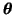. The cost function measures the misfit between the actual posterior pdf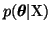and its approximation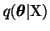.

The posterior is approximated as a product of independent Gaussian distributions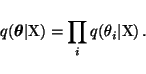(16)

Each individual Gaussian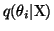is parametrised by the posterior mean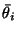and variance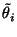of the parameter.

The functional form of the cost function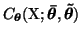is given in Chap. 6. The cost function can be interpreted to measure the misfit between the actual posteriorand its factorial approximation. It can also be interpreted as measuring the number of bits it would take to encode X when approximating the posterior pdf of the unknown variables by.

The cost function is minimised with respect to the posterior meansand variancesof the unknown variables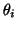. The end result of the learning is therefore not just an estimate of the unknown variables, but a distribution over the variables.

The simple factorising form of the approximationmakes the cost function computationally tractable. The cost function can be split into two terms, Cq and Cp, where the former is an expectation over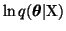and the latter is an expectation over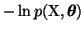.

It turns out that the term Cq is not a function of the posterior meansof the parameters, only the posterior variances. It has a similar term for each unknown variable.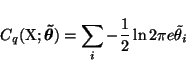(17)

Most of the terms of Cp are also trivial. The Gaussian densities in (8)-(15) yield terms of the form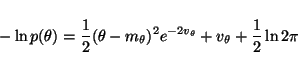(18)

Since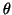,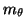and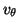are independent in q, the expectation over q yields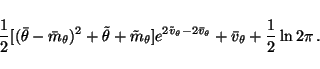(19)

Only the term originating from (7) needs some elaboration. Equation (7) yields(20)

and the expectation over q is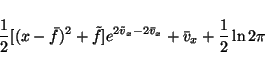(21)

The rest of this section is dedicated on evaluating the posterior mean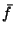and variance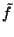of the function f. We shall begin from the sources and weights and show how the posterior mean and variance can be propagated through the network yielding the needed posterior mean and variance of the function f at the output. The effect of nonlinearities g of the hidden neurons are approximated by first and second order Taylor's series expansions around the posterior mean. Apart from that, the computation is analytical.

The function f consists of two multiplications with matrices and a nonlinearity in between. The posterior mean and variance for a product u = yz are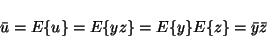(22)

and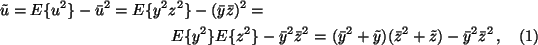given that y and z are posteriorly independent. According to the assumption of the factorising form of, the sources and the weights are independent and we can use the above formulas. The inputs going to hidden neurons consist of sums of products of weights and sources, each posteriorly independent, and it is therefore easy compute the posterior mean and variance of the inputs going to the hidden neurons; both the means and variances of a sum of independent variables add up.

Let us now pick one hidden neuron having nonlinearity g and input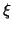, i.e., the hidden neuron is computing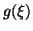. At this point we are not assuming any particular form of g although we are going to use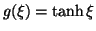in all the experiments; the following derivation is general and can be applied to any sufficiently smooth function g.

In order to be able to compute the posterior mean and variance of the function g, we are going to apply the Taylor's series expansion around the posterior mean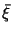of the input. We choose the second order expansion when computing the mean and the first order expansion when computing the variance. The choice is purely practical; higher order expansions could be used as well but these are the ones that can be computed from the posterior mean and variance of the inputs alone.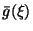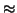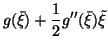(23)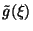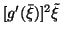(24)

After having evaluated the outputs of the nonlinear hidden neurons, it would seem that most of the work has already been done. After all, it was already shown how to compute the posterior mean and variance of a weighted sum and the outputs of the network will be weighted sums of the outputs of the hidden neurons. Unfortunately, this time the terms in the sum are no longer independent. The sources are posteriorly independent by virtue of the approximation, but the values of the hidden neurons are posteriorly dependent which enforces us to use a more complicated scheme for computing the posterior variances of these weighted sums. The posterior means will be as simple as before, though.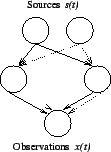The reason for the outputs of the hidden neurons to be posteriorly dependent is that the value of one source can potentially affect all the outputs. This is illustrated in Fig. 4. Each source affects the output of the whole network through several paths and in order to be able to determine the variance of the outputs, the paths originating from different sources need to be kept separate. This is done by keeping track of the partial derivatives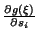. Equation (26) shows how the total posterior variance of the outputof one of the hidden neurons can be split into terms originating from each source plus a term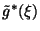which contains the variance originating from the weights and biases, i.e., those variables which affect any one output through only a single path.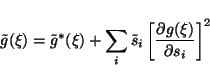(25)

When the outputs are multiplied by weights, it is possible to keep track of how this affects the posterior mean, the derivatives w.r.t. the sources and the variance originating from other variables than the sources, i.e., from weights and biases. The total variance of the output of the network is then obtained by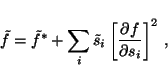(26)

where f denotes the components of the output and we have computed the posterior variance of the outputs of the network which is needed in (21). To recapitulate what is done, the contributions of different sources to the variances of the outputs of the network are monitored by computing the Jacobian matrix of the outputs w.r.t. the sources and keeping this part separate from the variance originating from other variables.

The only approximations done in the computation are the ones approximating the effect of nonlinearity. If the hidden neurons were linear, the computation would be exact. The nonlinearity of the hidden neurons is delt with by linearising around the posterior mean of the inputs of the hidden neurons. The smaller the variances the more accurate this approximation is. With increasing nonlinearity and variance of the inputs, the approximation gets worse.

Compared to ordinary forward phase of an MLP network, the computational complexity is greater by about a factor of 5N, where N is the number of sources. The factor five is due to propagating distributions instead of plain values. The need to keep the paths originating from different sources separate explains the factor N. Fortunately, much of the extra computation can be made into good use later on when adapting the distributions of variables.Next: Update Rules Up: Nonlinear Factor Analysis Previous: Definition of the Model
Harri Lappalainen
2000-03-03